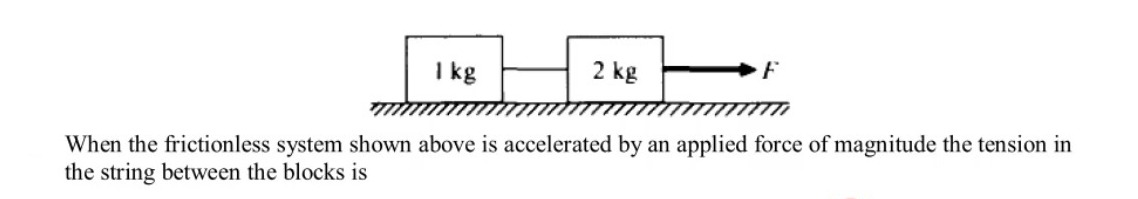# CU ATS Physics

## CU ATS Physics

1. A pulling force of 150 N making an angle of 53° to the horizontal is applied on a block of mass 15 kg. If the coefficients of static and kinetic friction between the surfaces are 0.60 and 0.50, respectively. What is the magnitude of acceleration of this block? (Given g = 9.8 m / $s^2)$
2. An object of mass 0.8 kg is projected vertically upwards with a velocity of 4.0 m/s at a height of 2.4 m above a tank that is filled with sand. It penetrates into the sand in the tank through a distance of 10 cm before coming to rest. How much work done by the resistance is offered by the sand? (Given g = 9.8 m/$s^2$)
3. The frequency of the third harmonic of a tube open at one end is 380 Hz. If the speed of the sound at that moment is 360 m/s, what is the length of the tube?
4. Two infinitely long parallel wires separated by a distance of 1.0 m as shown in the figure below. The wires carrying current of 6.0 A and 2.0 A placed in the same direction. What would be the resultant magnetic field at a distance of 0.5 m away from wire P?
5. Which of the following transitions in a hydrogen-like atom absorbs a photon with the longest wavelength?
6. When the frictionless system shown above is accelerated by an applied force of magnitude the tension in the string between the blocks is7. A force F directed at an angle θ above the horizontal is used to pull a crate a distance D across a level floor. The work done by the force F is
8. A 3 kg block with initial speed 4 m/s slides across a tough horizontal floor before coming the rest. The frictional force acting on the block is 3 N. How far does the block slide before coming to rest?
9. A 2-kilogram block rests at the edge of a platform that is 10 meters above level ground. The block is launched horizontally from the edge of the platform with an initial speed of 3 meters per second. Air resistance is negligible. The time it will take for the block to reach the ground is most nearly
10. An astronaut in an orbiting space craft attaches a inass $m$ to a string and whirls it around in uniform circular motion. The radius of the circle is $r$, the speed of the mass is $v$, and the tension in the string is $F$. If the mass, radius, and speed were all to double the tension required to maintain uniform circular motion would be
CU ATS Physics
You got {{userScore}} out of {{maxScore}} correct
{{title}}
{{image}}
{{content}}